# Designing a Hirth Spline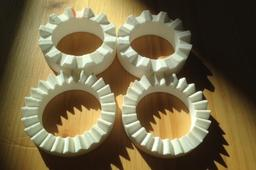Solidworks + Excel instruction on how to design a Hirth Spline.
Background on Hirth Splines: https://en.wikipedia.org/wiki/Hirth_joint

The basic idea of a Hirth spline / Hirth Joint is that the whole geometry is defined from a combination of a single reference point and a grinding/cutting geometry. Two Hirth splines with the same basic tooth geometry will match to form a secure joint that can transfer torque and allows for accurate alignment of parts.

Hirth splines and joints are often used in turbomachinery to accurately align various shaft parts and transfer torque.

1. ### Step 1: Offset Cutting Angle

First step is to calculate the cutting angle offset. Imagine that we start with a bar of material. And we want to cut a tooth at an angle to the radial cross sectional plane.

First we decide how many tooth we want, say 24, then we decide on the tooth angle, say 60 deg.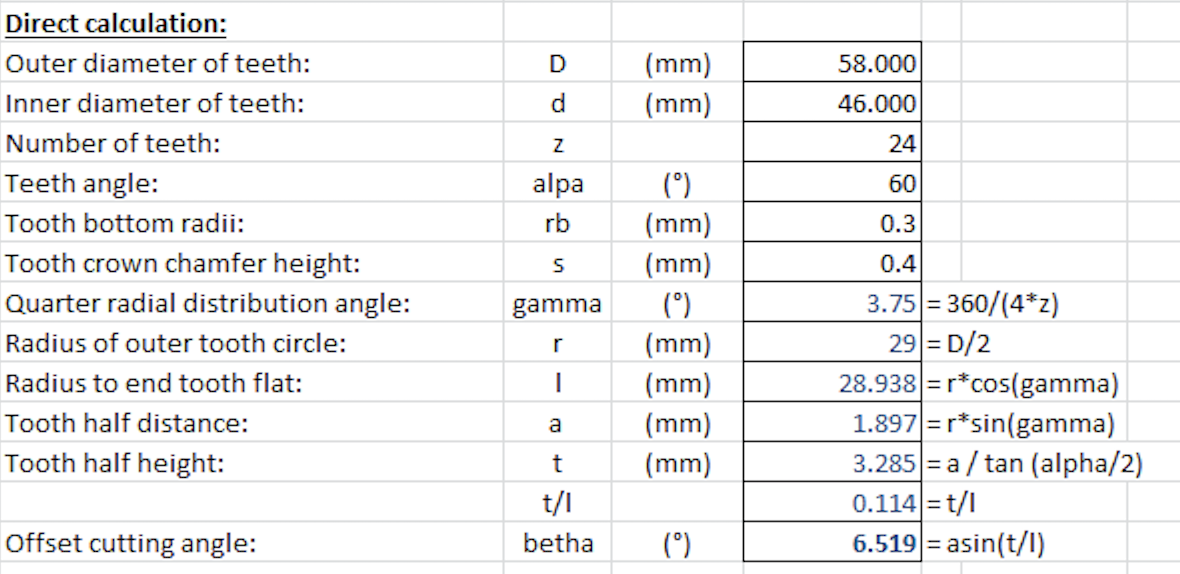This gets us an offset cutting angle of 6.519 degrees (decimal notation)

2. ### Step 2: Angle Notation

Note that there are two ways of communicating angles, in decimal form, like 6.519 deg. which means that it is 6 degrees and 0.519 part of 1 degree.

Alternatively you can use degrees,arc minutes(') and arc seconds("). In this method, the degrees are still written as normal, 6 degrees, however the additional fraction is based on 60 arc minutes in one degree and 60 arc seconds in one arc minute.

Hence 6.519 deg is the same as 6.31' (allowing for rounding)

To get from 6.31' to decimal notation (as used by Solidworks default) you divide the ' value by 60 to get back to the decimal notation, hence it becomes 0.5167, add the degree value and you get back to 6.5167 degrees in decimal notation.

Be carefull how suppliers of Hirth Splines/Joins note their angles, some use the decimal notation and others use the arc minute alternative.

3. ### Step 3: Modeling in Solidworks

Start off with modelling a bar or tube that represents your shaft: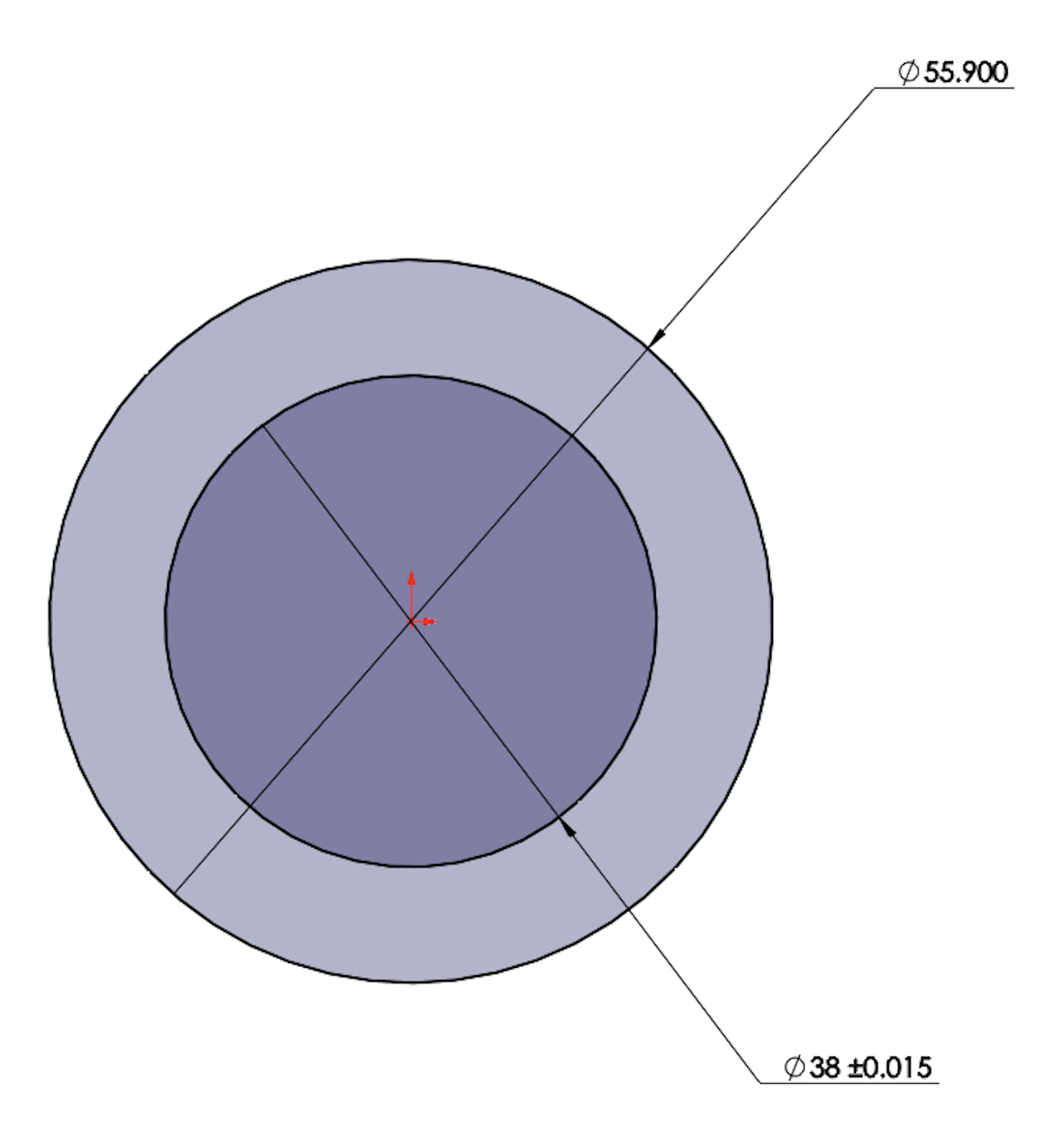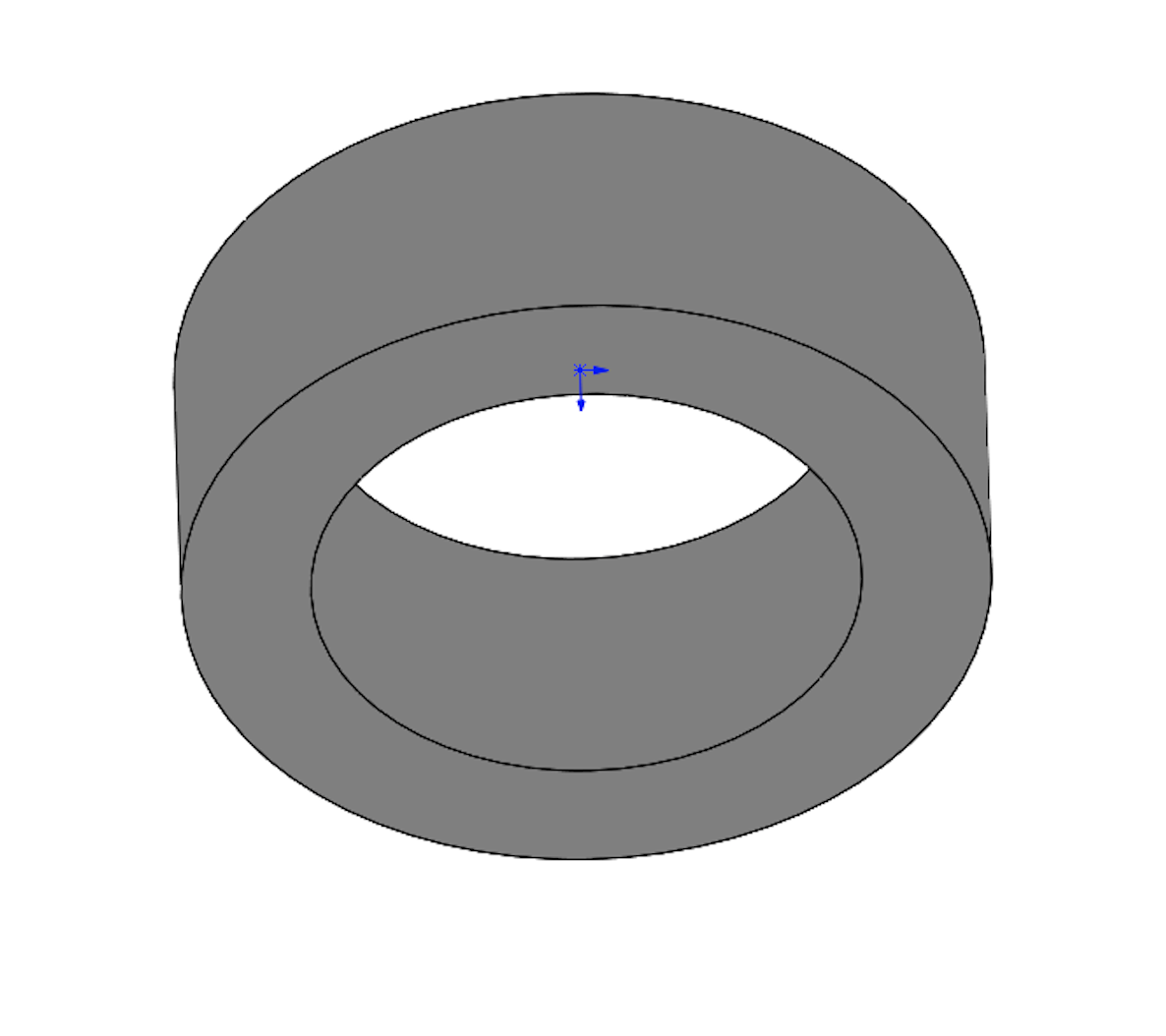Next we need to define the origin point and a line to reference the offset cutting plane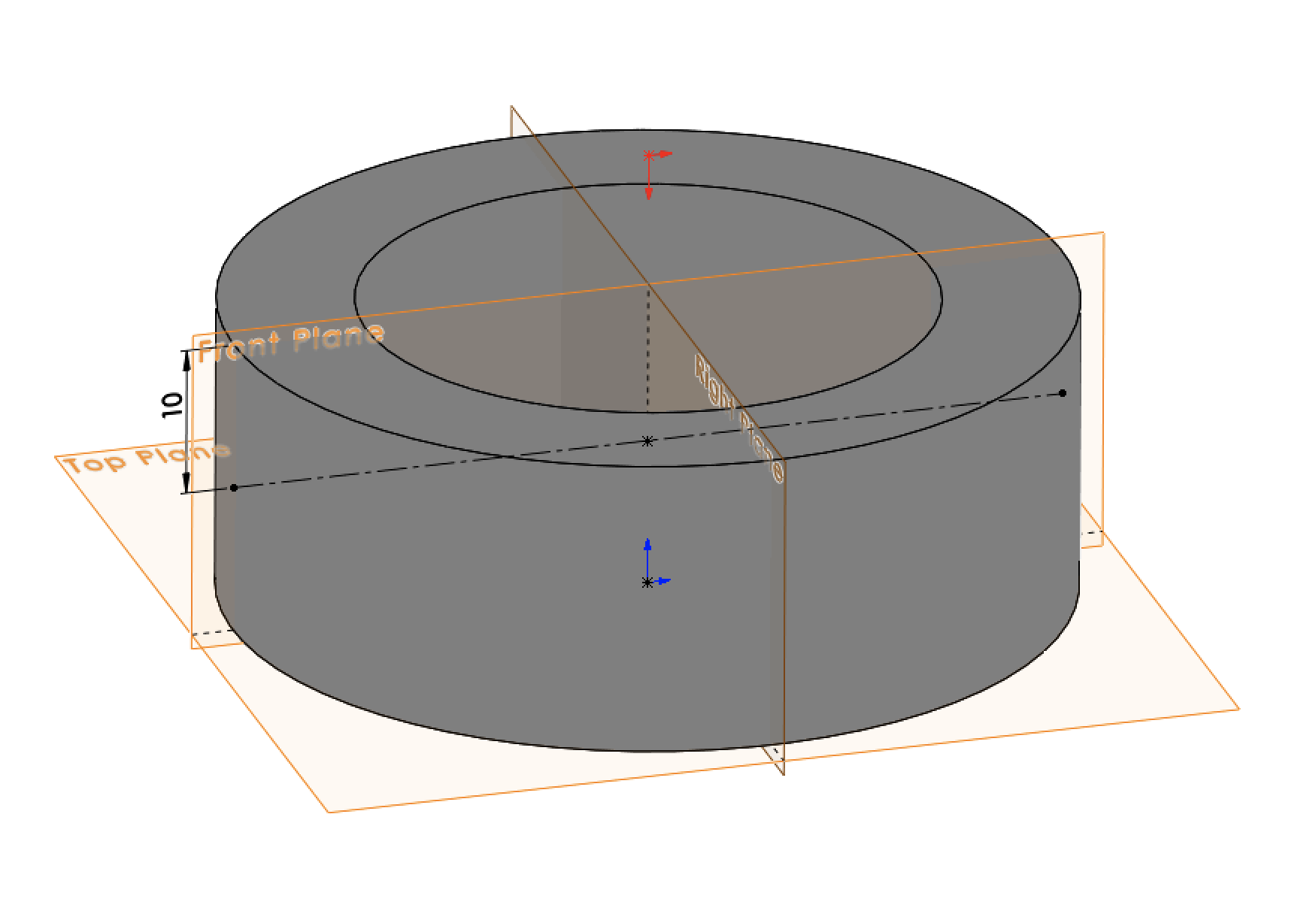Now we inert a reference plane with an angle offset as shown. We want an offset of 6.517 deg, if we reference the top surface of our shaft then we have to add 90 deg to the offset, so the value becomes 96.517 deg.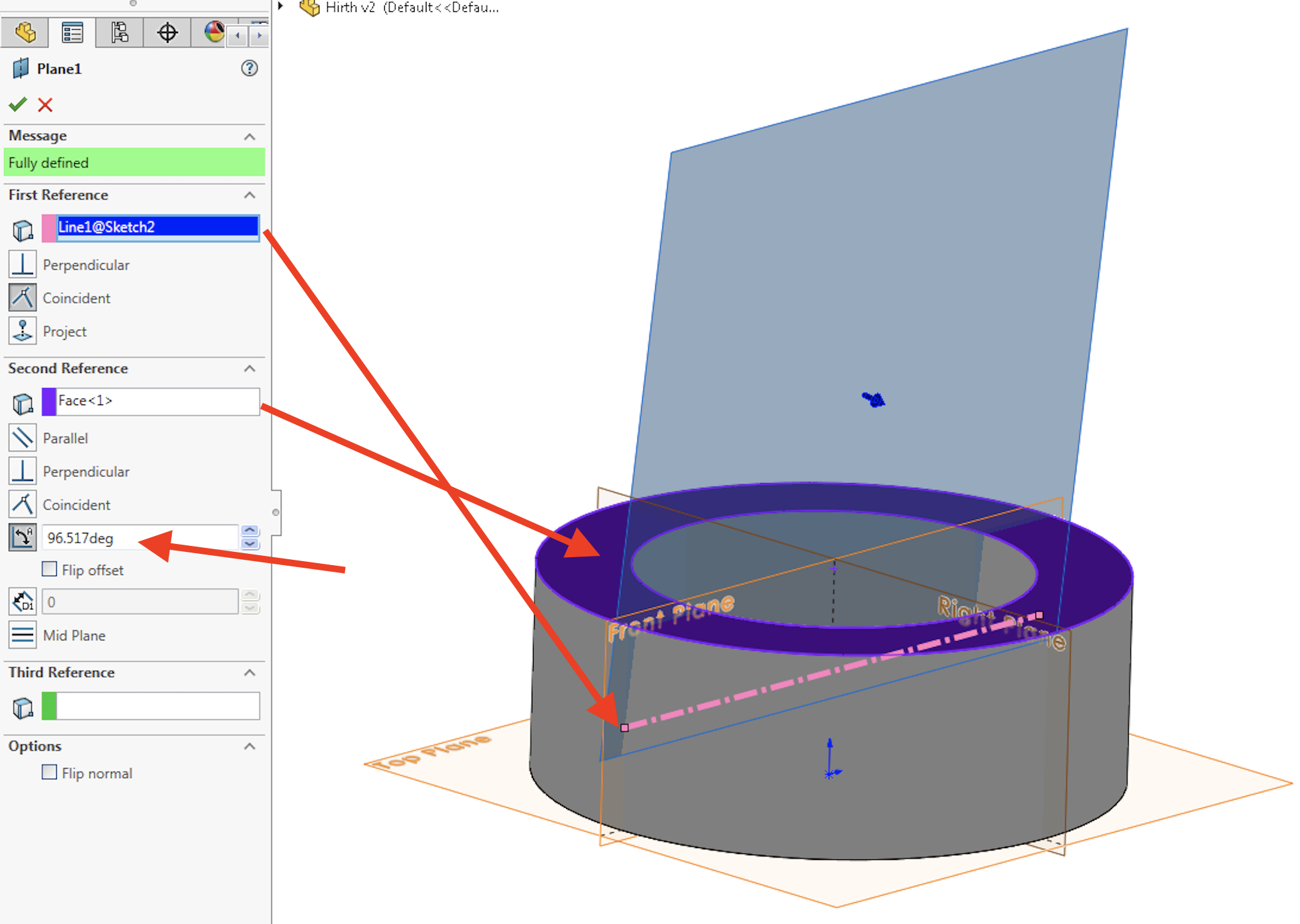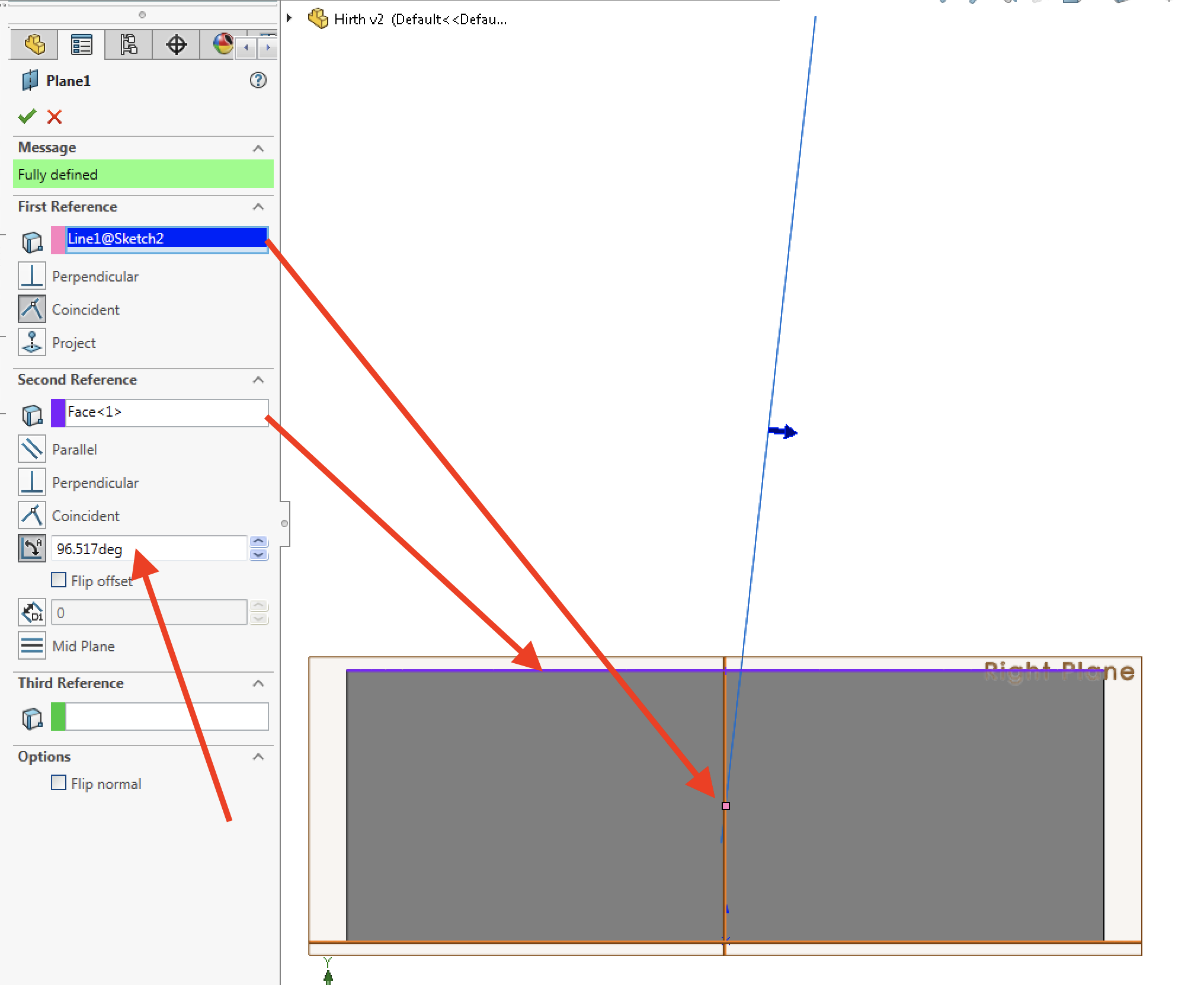On the reference plane we sketch the profile of the material we want to take away. Easiest to think about it is as if we sketch the profile of the grinding wheel that cuts the tooth. In this case a 60 deg angle, with a small radii at the tip.

Important! The tip of the triangle is at the origin point.We then perform the first cut: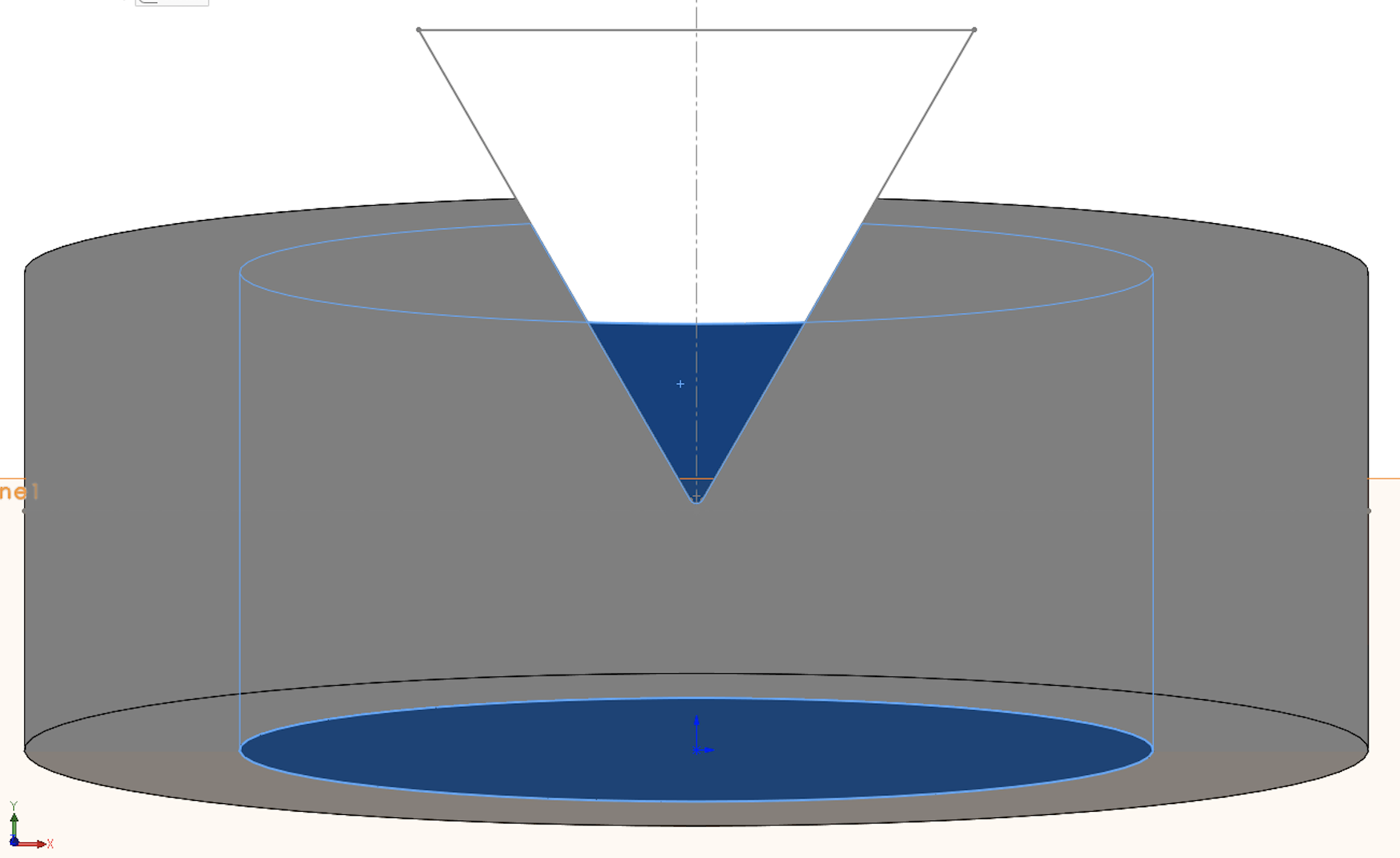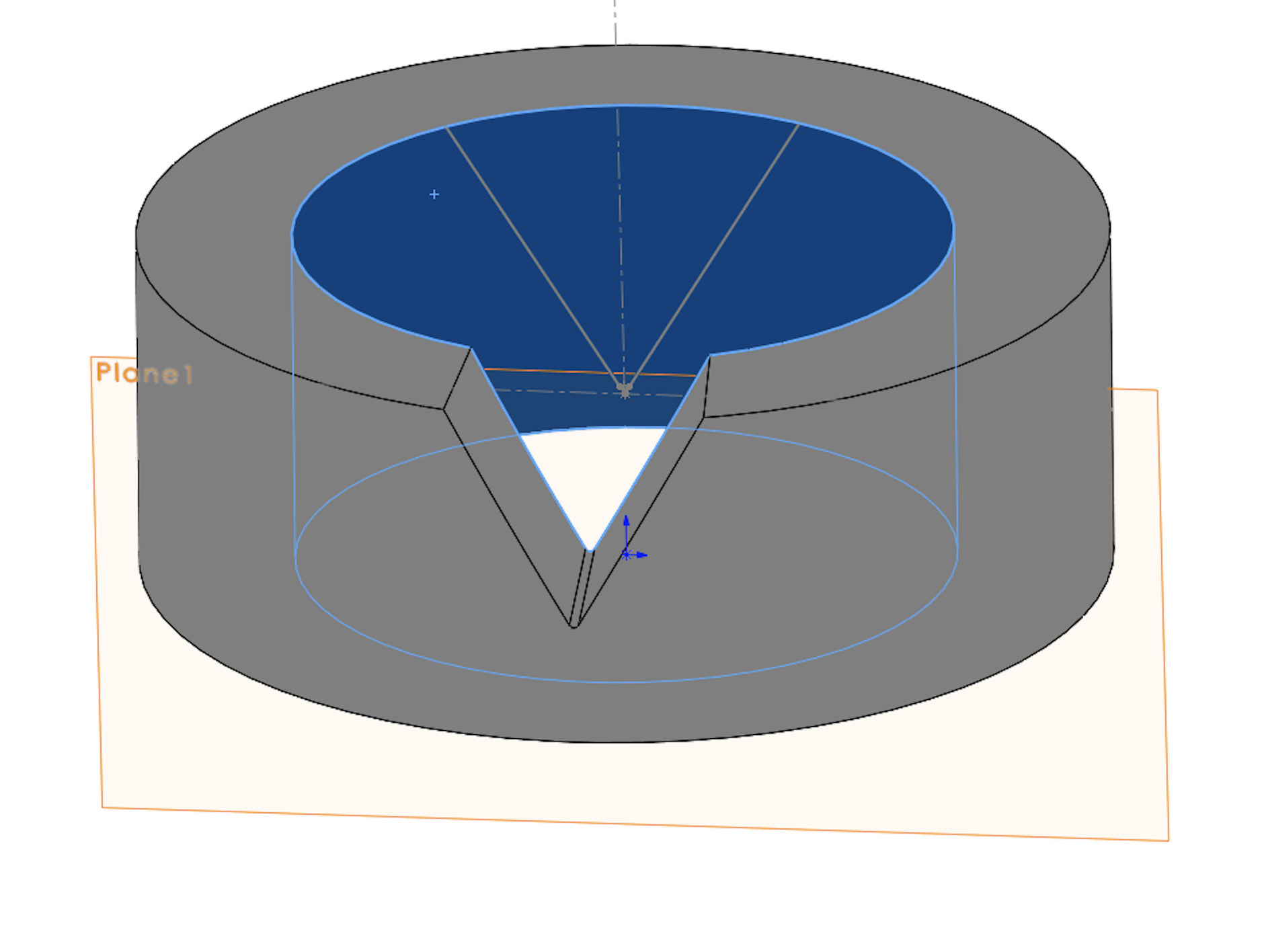Now perform a circular pattern 24 times: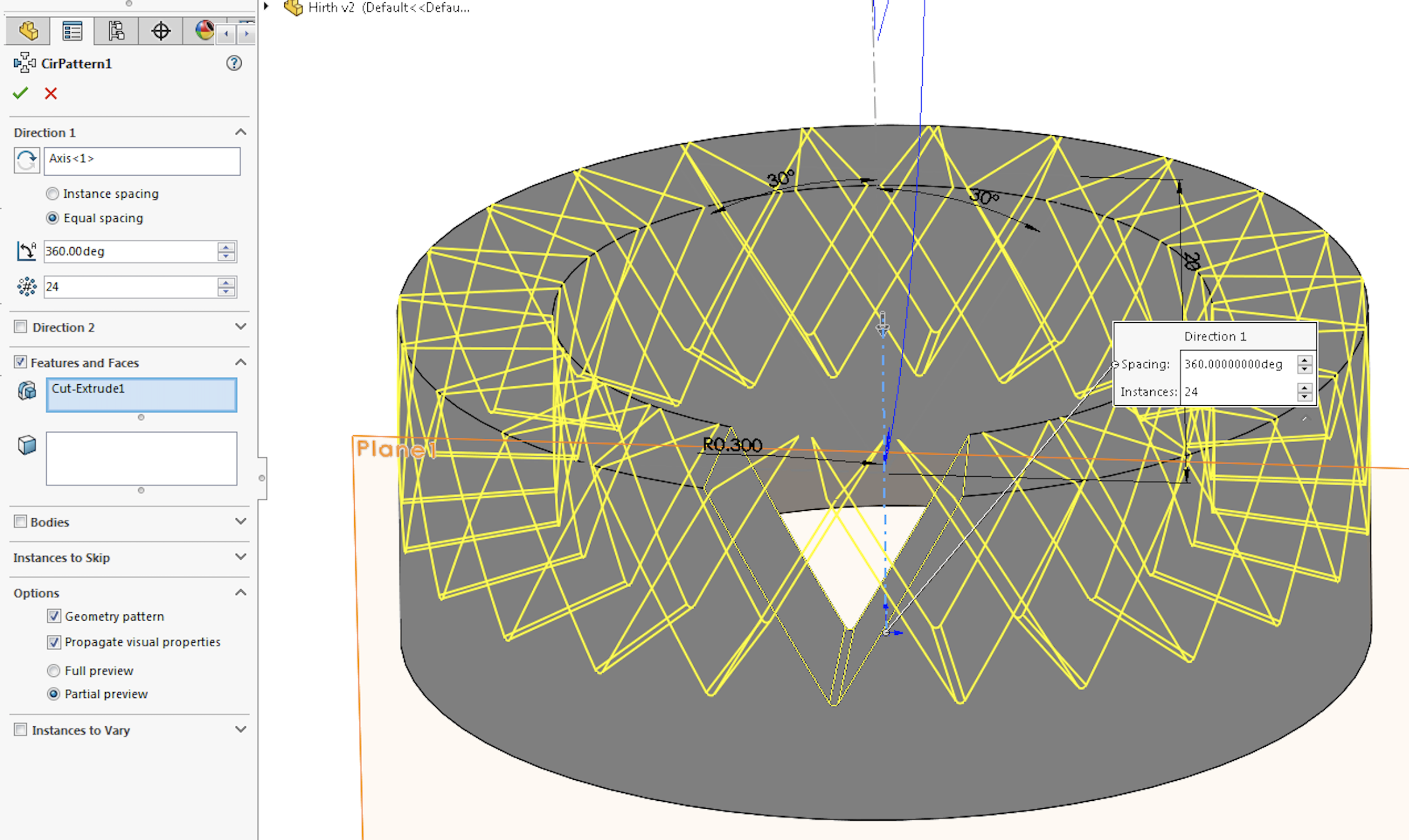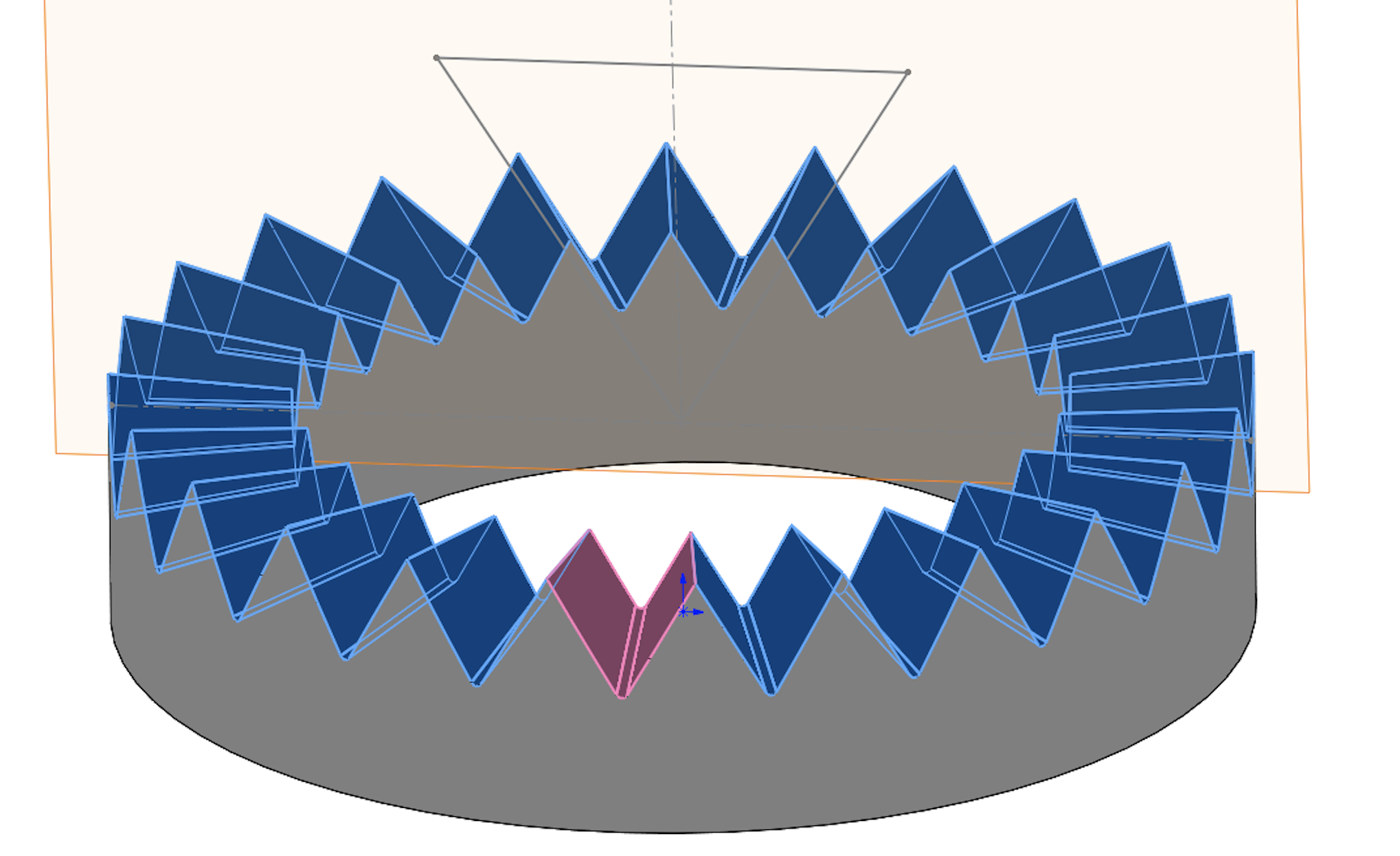Now it is almost complete,

Because we have a tooth root radius the Hirth spline won't fit to an identical Hirth spline yet. We need to machine away the tooth tips to create a clearance between the tooth tip and tooth root.

This is achieved by performing a Cut-revolve in Solidworks, and an equivalent turning operation during machining.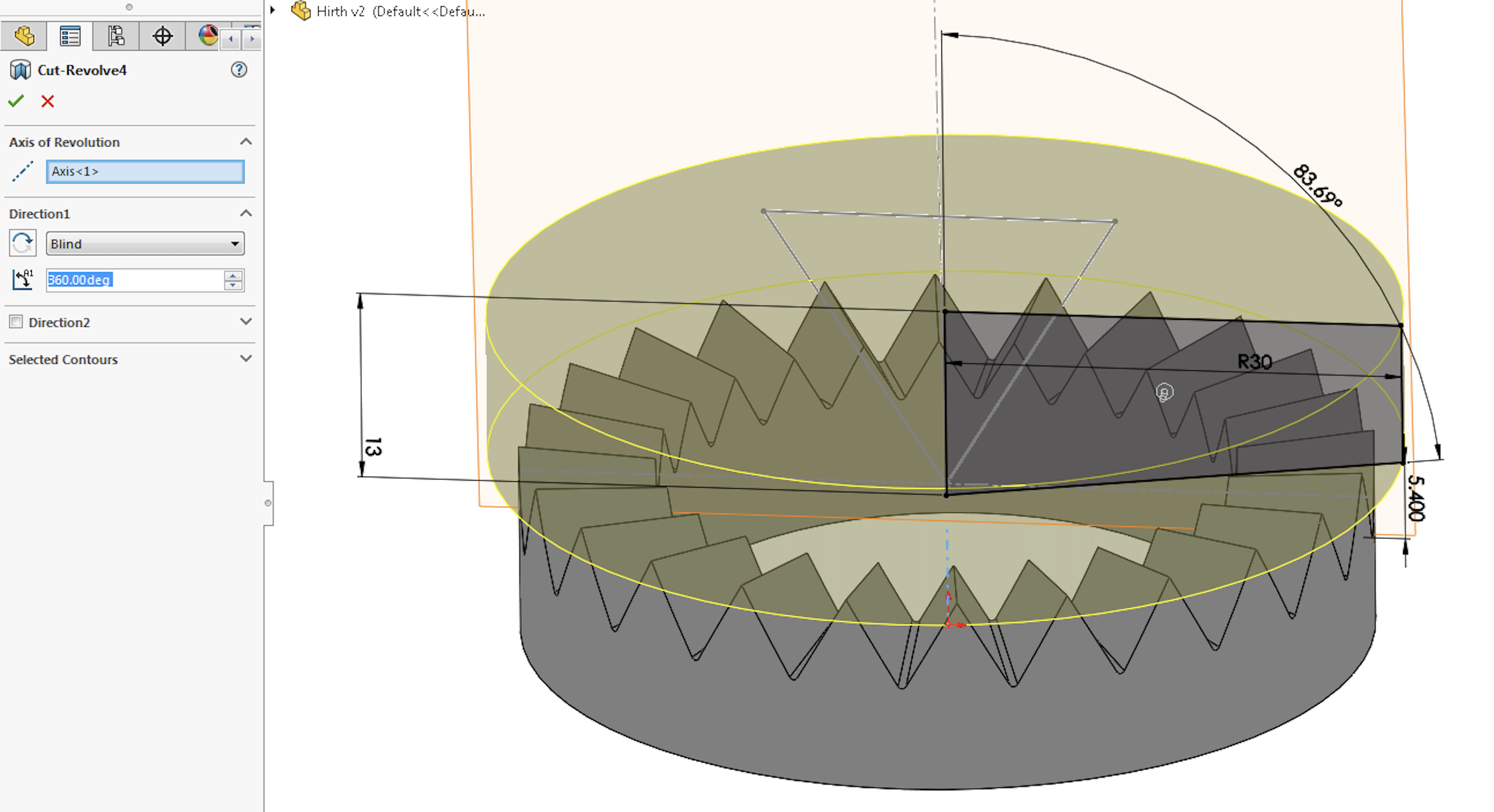4. ### Step 4: Final Check

Now we need to check the final geometry of the Hirth Spline by matching it up to an identical Hirth spline: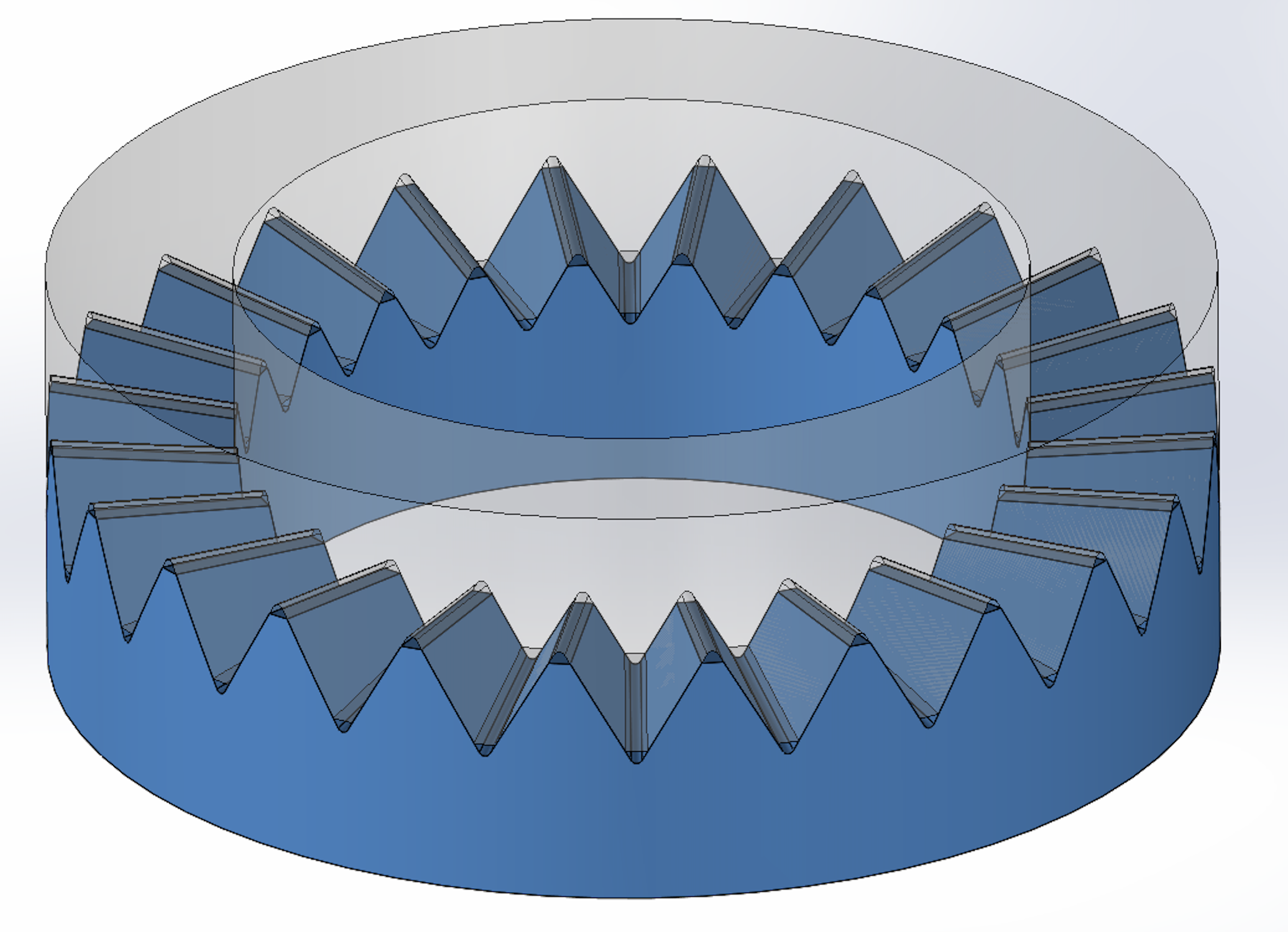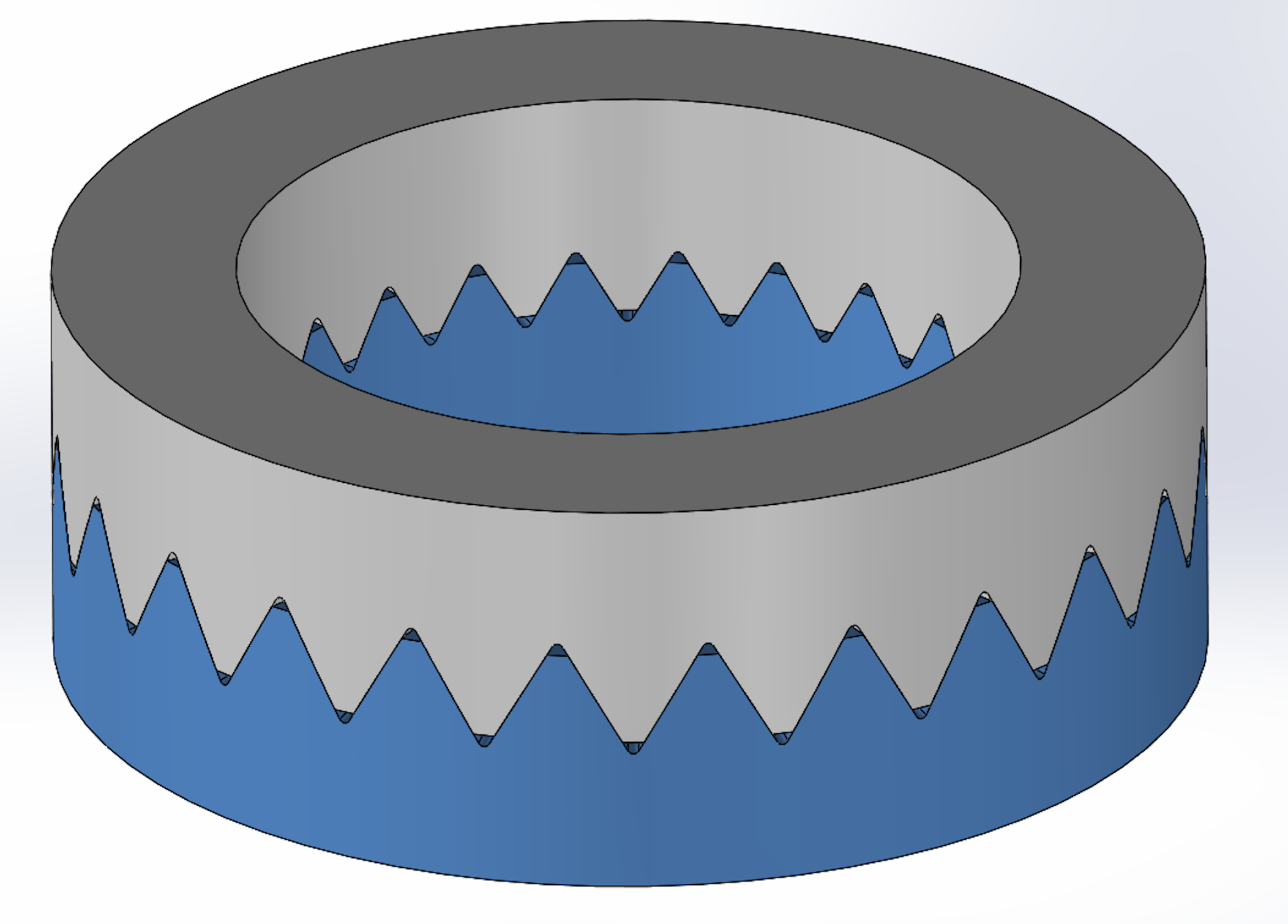### Comments

Please log in to add comments